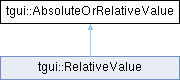TGUI  0.9.1
tgui::AbsoluteOrRelativeValue Class Reference

Class to store the a value that is either a constant or a ratio. More...

`#include <TGUI/AbsoluteOrRelativeValue.hpp>`

Inheritance diagram for tgui::AbsoluteOrRelativeValue:## Public Member Functions

TGUI_CONSTEXPR AbsoluteOrRelativeValue ()
Default constructor.

template<typename T , typename = typename std::enable_if<std::is_arithmetic<T>::value, T>::type>
TGUI_CONSTEXPR AbsoluteOrRelativeValue (T constant)
Constructor to set constant. More...

AbsoluteOrRelativeValue (const char *expression)
Construct the value from a string that either contains a value or a percentage. More...

AbsoluteOrRelativeValue (const String &expression)
Construct the value from a string that either contains a value or a percentage. More...

TGUI_CONSTEXPR float getValue () const
Returns the value. More...

TGUI_CONSTEXPR float getRatio () const
Returns the stored ratio. More...

TGUI_CONSTEXPR bool isConstant () const
Returns whether the value is constant or a ratio. More...

## Detailed Description

Class to store the a value that is either a constant or a ratio.

You don't have to explicitly create an instance of this class, numbers and strings are implicitly cast.

## ◆ AbsoluteOrRelativeValue() [1/3]

template<typename T , typename = typename std::enable_if<std::is_arithmetic<T>::value, T>::type>
 TGUI_CONSTEXPR tgui::AbsoluteOrRelativeValue::AbsoluteOrRelativeValue ( T constant )
inline

Constructor to set constant.

Parameters
 constant Value to set

## ◆ AbsoluteOrRelativeValue() [2/3]

 tgui::AbsoluteOrRelativeValue::AbsoluteOrRelativeValue ( const char * expression )
inline

Construct the value from a string that either contains a value or a percentage.

Parameters
 expression String to parse

## ◆ AbsoluteOrRelativeValue() [3/3]

 tgui::AbsoluteOrRelativeValue::AbsoluteOrRelativeValue ( const String & expression )
inline

Construct the value from a string that either contains a value or a percentage.

Parameters
 expression String to parse

## ◆ getRatio()

 TGUI_CONSTEXPR float tgui::AbsoluteOrRelativeValue::getRatio ( ) const
inline

Returns the stored ratio.

Returns
The ratio that is multiplied with the parent size to get the value, when the value isn't a constant

## ◆ getValue()

 TGUI_CONSTEXPR float tgui::AbsoluteOrRelativeValue::getValue ( ) const
inline

Returns the value.

Returns
The constant value or the value based on the given ratio and parent size

## ◆ isConstant()

 TGUI_CONSTEXPR bool tgui::AbsoluteOrRelativeValue::isConstant ( ) const
inline

Returns whether the value is constant or a ratio.

Returns
Does the value contain a constant?

The documentation for this class was generated from the following file: